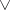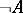# Law of the excluded middle

(diff) ← Older revision | Latest revision (diff) | Newer revision → (diff)
The law in classical logic stating that one of the two statements "A" or "not A" is true. The law of the excluded middle is expressed in mathematical logic by the formula, wheredenotes disjunction anddenotes negation. From the intuitionistic (constructive) point of view, establishing the truth of a statementmeans establishing the truth of eitheror. Since there is no general method for establishing in a finite number of steps the truth of an arbitrary statement, or of that of its negation, the law of the excluded middle was subjected to criticism by representatives of the intuitionistic and constructive directions in the foundations of mathematics (cf. Intuitionism; Constructive mathematics).